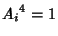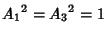## Cycle Graph

A cycle of a Graph is a subset of the Edge-set of the Graph which forms a Chain (Graph), the first node of which is also the last. This type of cycle is also called a Circuit.A cycle graph is a Graph which shows cycles of a Group as well as the connectivity between the cycles. Several examples are shown above. For Finite Group Z4, the group elementssatisfy, where 1 is the Identity Element, and two elements satisfy.

For a Cyclic Group of Composite Order(e.g., Finite Group Z4, Finite Group Z6, Finite Group Z8), the degenerate subcycles corresponding to factors dividingare often not shown explicitly since their presence is implied.

See also Chain (Graph), Characteristic Factor, Cyclic Graph, Cyclic Group, Hamiltonian Cycle, Walk

References

Shanks, D. Solved and Unsolved Problems in Number Theory, 4th ed. New York: Chelsea, pp. 83-98, 1993.